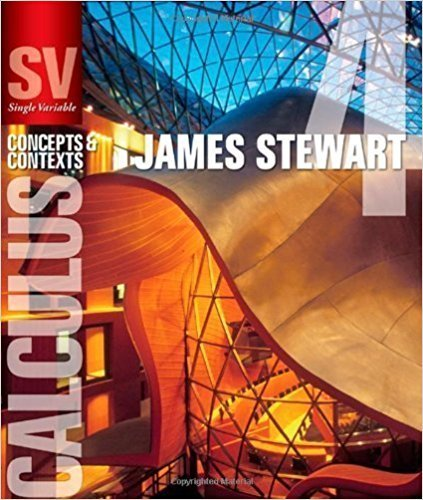×
×

# Solutions for Chapter 6.6: APPLICATIONS TO PHYSICS AND ENGINEERING## Full solutions for Single Variable Calculus: Concepts and Contexts (Stewart's Calculus Series) | 4th Edition

ISBN: 9780495559726Solutions for Chapter 6.6: APPLICATIONS TO PHYSICS AND ENGINEERING

Solutions for Chapter 6.6
4 5 0 351 Reviews
11
5
##### ISBN: 9780495559726

Chapter 6.6: APPLICATIONS TO PHYSICS AND ENGINEERING includes 52 full step-by-step solutions. Since 52 problems in chapter 6.6: APPLICATIONS TO PHYSICS AND ENGINEERING have been answered, more than 20983 students have viewed full step-by-step solutions from this chapter. Single Variable Calculus: Concepts and Contexts (Stewart's Calculus Series) was written by and is associated to the ISBN: 9780495559726. This textbook survival guide was created for the textbook: Single Variable Calculus: Concepts and Contexts (Stewart's Calculus Series), edition: 4. This expansive textbook survival guide covers the following chapters and their solutions.

Key Calculus Terms and definitions covered in this textbook
• Aphelion

The farthest point from the Sun in a planet’s orbit

• Arc length formula

The length of an arc in a circle of radius r intercepted by a central angle of u radians is s = r u.

• Binomial probability

In an experiment with two possible outcomes, the probability of one outcome occurring k times in n independent trials is P1E2 = n!k!1n - k2!pk11 - p) n-k where p is the probability of the outcome occurring once

• Compounded k times per year

Interest compounded using the formula A = Pa1 + rkbkt where k = 1 is compounded annually, k = 4 is compounded quarterly k = 12 is compounded monthly, etc.

• Conic section (or conic)

A curve obtained by intersecting a double-napped right circular cone with a plane

• Divergence

A sequence or series diverges if it does not converge

• Explicitly defined sequence

A sequence in which the kth term is given as a function of k.

• Graph of a function ƒ

The set of all points in the coordinate plane corresponding to the pairs (x, ƒ(x)) for x in the domain of ƒ.

• Interquartile range

The difference between the third quartile and the first quartile.

• Invertible linear system

A system of n linear equations in n variables whose coefficient matrix has a nonzero determinant.

• Line of travel

The path along which an object travels

• LRAM

A Riemann sum approximation of the area under a curve ƒ(x) from x = a to x = b using x1 as the left-hand endpoint of each subinterval

• Measure of center

A measure of the typical, middle, or average value for a data set

• Pythagorean identities

sin2 u + cos2 u = 1, 1 + tan2 u = sec2 u, and 1 + cot2 u = csc2 u

• Slope-intercept form (of a line)

y = mx + b

• Solve an equation or inequality

To find all solutions of the equation or inequality

• Solve by substitution

Method for solving systems of linear equations.

• Sum of two vectors

<u1, u2> + <v1, v2> = <u1 + v1, u2 + v2> <u1 + v1, u2 + v2, u3 + v3>

• y-axis

Usually the vertical coordinate line in a Cartesian coordinate system with positive direction up, pp. 12, 629.

• Zero factorial

See n factorial.

×